## ML Aggarwal Class 6 Solutions for ICSE Maths Chapter 15 Data Handling Objective Type Questions

Mental Maths
Question 1.
Fill in the blanks:
(i) A ……. is a collection of numerical figures to give some information.
(ii) Each numerical figure in a data is called an ………..
(iii) The number of times a particular observation occurs in a data is called … of the observation.
(iv) A data arranged in ascending or descending order is called an ………… data.
(v) A pictorial representation of a data is called a …………
(vi) If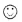represent 5 students thenrepresent ………… students.
(vii) In a pictograph, if one bicycle represents 20 bicycles then 140 bicycles can be represented by ………… bicycles.
(viii)In a pictograph, if one electric bulb represents 10 bulbs then the picture of half a bulb will represent ………… bulbs.
(ix) In a pictograph, if the symbol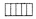represents 50 fruits baskets, thenrepresent ………… fruit baskets.
(x) The frequency of 8 is written symbolically as ………… using tally marks.
(xi) In a bar graph, the bars are of uniform …………
(xii) The mean of the first 8 natural numbers is …………
Solution:
(i) A data is a collection of numerical figures to give some information.
(ii) Each numerical figure in a data is called an observation.
(iii) The number of times a particular observation
occurs in a data is called frequency of the observation.
(iv) A data arranged in ascending or descending order
is called an arrayed data.
(v) A pictorial representation of a data is called a pictograph.
(vi) Ifrepresent 5 students then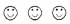represent 15 students.
(vii) In a pictograph, if one bicycle represents 20 bicycles
then 140 bicycles can be represented by 7 bicycles.
(viii)In a pictograph, if one electric bulb represents 10 bulbs
then the picture of half a bulb will represent 5 bulbs.
(ix) In a pictograph, if the symbolrepresents 50 fruits baskets,
then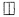represent 120 fruit baskets.
(x) The frequency of 8 is written symbolically
asusing tally marks.
(xi) In a bar graph, the bars are of uniform width.
(xii) The mean of the first 8 natural numbers is 4.5

Question 2.
State whether the following statements are true (T) or false (F):
(i) If the data is large, it is difficult to get information from raw data.
(ii) Pictographs and bar graphs help us in understanding and analyzing a data.
(iii) In a bar graph, bars can be drawn either vertically or horizontally.
(iv) In a bar graph, width of a bar has no significance. It is only for eye attraction.
(v) Usually, the tally marks are recorded in bunches of 5.
(vi) The frequency 9 is represented as filtlll using tally marks.
(vii) Mentioning of scale is necessary in pictographs.
(viii) Mean is always one of the number in a given data.
(ix) Median is always one of the numbers in a given data.
Solution:
(i) If the data is large, it is difficult to get information
from raw data. True
(ii) Pictographs and bar graphs help us in understanding
and analyzing a data. True
(iii) In a bar graph, bars can be drawn either vertically
or horizontally. True.
(iv) In a bar graph, width of a bar has no significance.
It is only for eye attraction. True
(v) Usually, the tally marks are recorded in bunches of 5. True
(vi) The frequency 9 is represented as fMI using tally marks. False
(vii) Mentioning of scale is necessary in pictographs. True
(viii) Mean is always one of the number in a given data. False
(ix) Median is always one of the numbers in a given data. False

Multiple Choice Questions
Observe the following pictograph which shows the number of ice cream cones sold by school ‘ canteen during a week. Chose the correct answer from the given options for questions 3 to 7:Question 3.
The minimum number of ice cream cones were sold on:
(a) Monday
(b) Saturday
(c) Tuesday
(d) Thursday
Solution:
Thursday (d)

Question 4.
The maximum number of ice cream cones were sold on:
(a) Tuesday
(b) Friday
(c) Wednesday
(d) Thursday
Solution:
Tuesday (a)

Question 5.
Ratio of the number of ice cream cones sold on Saturday to the number of ice cream cones sold on Wednesday is.
(a) 3 : 2
(b) 2 : 3
(c) 4 : 5
(d) 4 : 7
Solution:
Saturday = 8
Wednesday = 12
⇒ 8 : 12
⇒ 2 : 3 (b)

Question 6.
Total number of ice cream cones sold during the whole week was:
(a) 33
(b) 67
(c) 65
(d) 57
Solution:
Monday = 10
Tuesday = 16
Wednesday = 12
Thursday = 7
Friday = 14
Saturday = 8
Total 67 ice cream (b)

Question 7.
If the cost of one ice cream cone is ₹20, then the sale value on Thursday was:
(a) ₹70
(b) ₹100
(c) ₹140
(d) ₹1340
Solution:
Number of ice creams sold on Thursday = 7
= ₹20 × 7 = ₹140 (c)

Observe the adjoining bar graph, showing the number of one-day international mathces played by cricket teams of different countries. Choose the correct answer from the given four options for questions 8 to 11: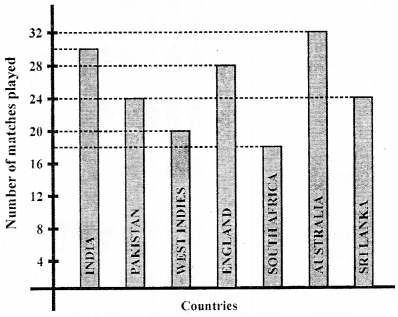Question 8.
Which country played maximum number of matches? .
(a) India
(b) England
(c) Pakistan
(d) Australia
Solution:
Australia (d)

Question 9.
How many matches did South Africa play?
(a) 16
(b) 18
(c) 20
(d) 24
Solution:
18 (b)

Question 10.
How many more matches were played by India than Pakistan?
(a) 6
(b) 12
(c) 24
(d) 30
Solution:
Pakistan = 24
India = 30
∴ 30 – 24 = 6 (a)

Question 11.
Ratio of the number of matches played by India to the number of matches played by Sri Lanka is
(a) 4 : 5
(b) 5 : 4
(c) 4 : 3
(d) 7 : 6
Solution:
India = 30
Sri Lanka = 24
⇒ 30 : 24 = 5 : 4 (b)

Choose the correct answer from the given four options for questions 12 and 13:
Question 12.
The mean of the first 6 odd natural numbers is
(a) 5
(b) 5.5
(c) 6
(d) 6.5
Solution:
First 6 odd natural numbers are:
1, 3, 5, 7, 9, 11
1 + 3 + 5 + 7 + 9 + 11
Mean = $$\frac{1+3+5+7+9+11}{6}$$
= $$\frac{36}{6}=6$$ (c)

Question 13.
The median of the numbers 4, 4, 7, 5, 7, 6, 1, 3, 11 is
(a) 7
(b) 6
(c) 5
(d) 4
Solution:
Arrange the data in ascending order : 3, 4, 4, 5, 6, 7, 1,1, 11
Total terms = 9
Median = $$\left(\frac{n+1}{2}\right)$$th term
= $$\frac{9+1}{2}$$ = 4th term = 6 (b)